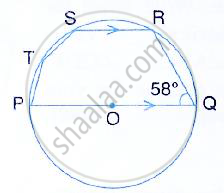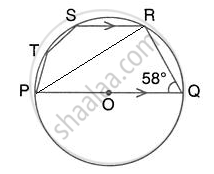Share

In the Given Figure, Pq is a Diameter. Chord Sr is Parallel to Pq. Given that ∠Pqr = 58°, Calculate: (I) ∠Rpq, (Ii) ∠Stp - ICSE Class 10 - Mathematics

ConceptArc and Chord Properties - Angle in a Semi-circle is a Right Angle

Question

In the given figure, PQ is a diameter. Chord SR is parallel to PQ. Given that ∠PQR = 58°,
Calculate:
(i) ∠RPQ, (ii) ∠STPSolutionJoin PR.
(i) ∠PRQ = 90°
(Angle in a semicircle)
∴ In right triangle PQR,
∠RPQ = 90° - ∠PQR = 90° - 58° = 32°

(ii) Also, SR || PQ
∠PRS = ∠RPQ = 32 (Alternate angles) In cyclic quadrilateral PRST,
∠STP =180° - ∠PRS = 180° - 32° = 148°
(pair of opposite angles in a cyclic quadrilateral are supplementary)

Is there an error in this question or solution?

Video TutorialsVIEW ALL 

Solution In the Given Figure, Pq is a Diameter. Chord Sr is Parallel to Pq. Given that ∠Pqr = 58°, Calculate: (I) ∠Rpq, (Ii) ∠Stp Concept: Arc and Chord Properties - Angle in a Semi-circle is a Right Angle.
S